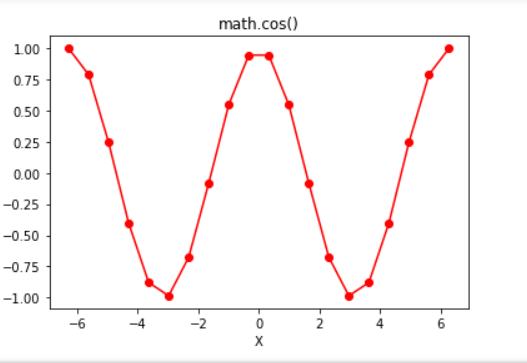# Python | math.cos() function

In Python, math module contains a number of mathematical operations, which can be performed with ease using the module. `math.cos()` function returns the cosine of value passed as argument. The value passed in this function should be in radians.

Syntax: math.cos(x)

Parameter:
x : value to be passed to cos()

Returns: Returns the cosine of value passed as argument

Code #1:

 `# Python code to demonstrate the working of cos() ` `    `  `# importing "math" for mathematical operations  ` `import` `math  ` `   `  `a ``=` `math.pi ``/` `6` `    `  `# returning the value of cosine of pi / 6  ` `print` `(``"The value of cosine of pi / 6 is : "``, end ``=``"")  ` `print` `(math.cos(a))  `

Output:

```The value of cosine of pi/6 is : 0.8660254037844387
```

Code #2:

 `# Python program showing  ` `# Graphical representation of  ` `# cos() function  ` `import` `math ` `import` `numpy as np ` `import` `matplotlib.pyplot as plt  ` ` `  `in_array ``=` `np.linspace(``-``(``2` `*` `np.pi), ``2` `*` `np.pi, ``20``) ` ` `  `out_array ``=` `[] ` ` `  `for` `i ``in` `range``(``len``(in_array)): ` `    ``out_array.append(math.cos(in_array[i])) ` `    ``i ``+``=` `1` ` `  `  `  `print``(``"in_array : "``, in_array)  ` `print``(``"\nout_array : "``, out_array)  ` ` `  `# red for numpy.sin()  ` `plt.plot(in_array, out_array, color ``=` `'red'``, marker ``=` `"o"``)  ` `plt.title(``"math.cos()"``)  ` `plt.xlabel(``"X"``)  ` `plt.ylabel(``"Y"``)  ` `plt.show()  `

Output:

in_array : [-6.28318531 -5.62179738 -4.96040945 -4.29902153 -3.6376336 -2.97624567
-2.31485774 -1.65346982 -0.99208189 -0.33069396 0.33069396 0.99208189
1.65346982 2.31485774 2.97624567 3.6376336 4.29902153 4.96040945
5.62179738 6.28318531]

out_array : [1.0, 0.7891405093963934, 0.2454854871407988, -0.40169542465296987, -0.8794737512064891, -0.9863613034027223, -0.6772815716257412, -0.08257934547233249, 0.5469481581224268, 0.9458172417006346, 0.9458172417006346, 0.5469481581224268, -0.0825793454723316, -0.6772815716257405, -0.9863613034027223, -0.8794737512064893, -0.40169542465296987, 0.2454854871407988, 0.7891405093963934, 1.0]Attention geek! Strengthen your foundations with the Python Programming Foundation Course and learn the basics.

To begin with, your interview preparations Enhance your Data Structures concepts with the Python DS Course.

My Personal Notes arrow_drop_upCheck out this Author's contributed articles.

If you like GeeksforGeeks and would like to contribute, you can also write an article using contribute.geeksforgeeks.org or mail your article to contribute@geeksforgeeks.org. See your article appearing on the GeeksforGeeks main page and help other Geeks.

Please Improve this article if you find anything incorrect by clicking on the "Improve Article" button below.

Article Tags :

1

Please write to us at contribute@geeksforgeeks.org to report any issue with the above content.